Zeitschriftendaten
Format
Zeitschrift
eISSN
2083-831X
Erstveröffentlichung
09 Nov 2012
Erscheinungsweise
4 Hefte pro Jahr
Sprachen
Englisch
Uneingeschränkter Zugang

# Numerical Evaluation of Partially Strengthened Floating Granular Pile Raft With Vertical and Radial Displacement Compatibility

###### Akzeptiert: 14 Feb 2022
Zeitschriftendaten
Format
Zeitschrift
eISSN
2083-831X
Erstveröffentlichung
09 Nov 2012
Erscheinungsweise
4 Hefte pro Jahr
Sprachen
Englisch
Introduction

In circumstances where a raft foundation solitary doesn’t fill the plan demands, piles are added with raft to improve its performance, which is called a piled raft, where the piles are involved as settlement easer. Piled raft foundation system has been most particularly applied to high-rise buildings all over the world and progressively recognized as a relatively economical system for bridges and large-scale industrial plants. At the place of the conventional piled raft system of foundation, the piles are replaced with the granular pile (GP)/stone column, and in the present study, the GP is strengthened in the upper portion. Strengthening means the parent material of GP is replaced partially by constructing pile with the granular material (e.g., gravel, pebbles, or coarse dense sand) for better stiffness and strength. The strengthening in the upper portion of the pile is done to overcome the problem of bulging in the upper portion of the GP. Strengthening is represented through two variables, viz. one is for comparative length of strengthening of GP and other is for comparative strengthening of the upper portion to lower unstrengthened portion of the GP. The impact of the factors has been studied on the overall settlement, the lateral bulging of the GPs, and load distribution between raft, pile, and pile base. The outcomes showed that the GPs in compressible soil reduce the settlement and the lateral displacement of the GPs effectively. Additional improvements in the settlement of the raft and in the lateral displacement also occurred due to the increase of the strengthening parameters.

Problem Definition

Partially strengthened floating granular piled raft foundation carrying an axial load F, with the length of GP, Lp, radius ‘a’, and diameter, dp =2a, is shown in Fig. 1. The compressible soil is represented by the modulus of deformation, Es, and Poisson’s ratio, υs. The GP is compressible with the modulus of deformation, Ep, and Poisson’s ratio, νp. The comparative strengthening of GP is defined as Kp= Ep/Es, the ratio of deformation moduli of the GP to that of the soil. In the present analysis, it is assumed that the top portion of length Lsr×Lp has been strengthened where λr is constant for comparative length of strengthening of GP. The modulus of the deformation of the partially strengthened portion is Estp. Comparative strengthening (Kstp) of the partially strengthened section of GP is μr times that of the unstrengthened portion, i.e., Kstp = μrKp, where μr is strengthening factor. It is assumed that the Poisson ratio νp is the same throughout the GP.

Mindlin’s expressions for vertical and horizontal displacements due to both vertical and horizontal point loads acting within the soil mass are used to get vertical and radial soil displacements of GP. The pile is discretized into ‘n’ cylindrical element (segments), and each segment is additionally subdivided vertically into ‘nz’ and circumferentially into ‘nt’ segments and GP base is separated into ‘nb’ annular and ‘nbt’ angular subdivisions for numerical integration. The settlements for cylindrical segments are intended at the nodes at the periphery of each segment on the GP–soil interface, while for the base, the node is at the center of GP.

The raft is supposed to be rigid and of diameter, dr. For mathematical integration, raft is discretized into ‘kr’ number of annular rings of equal areas. It is additionally subdivided into ‘kt’ number of angular subdivisions and ‘τ’ is the interface shear stresses between GP and soil, ‘pr’ is the contact pressures at the raft–soil interface and ‘pb’ is base pressure at pile tip as shown in Fig. 2. Settlement nodes are at the interfaces of soil and raft and the GP–soil interface.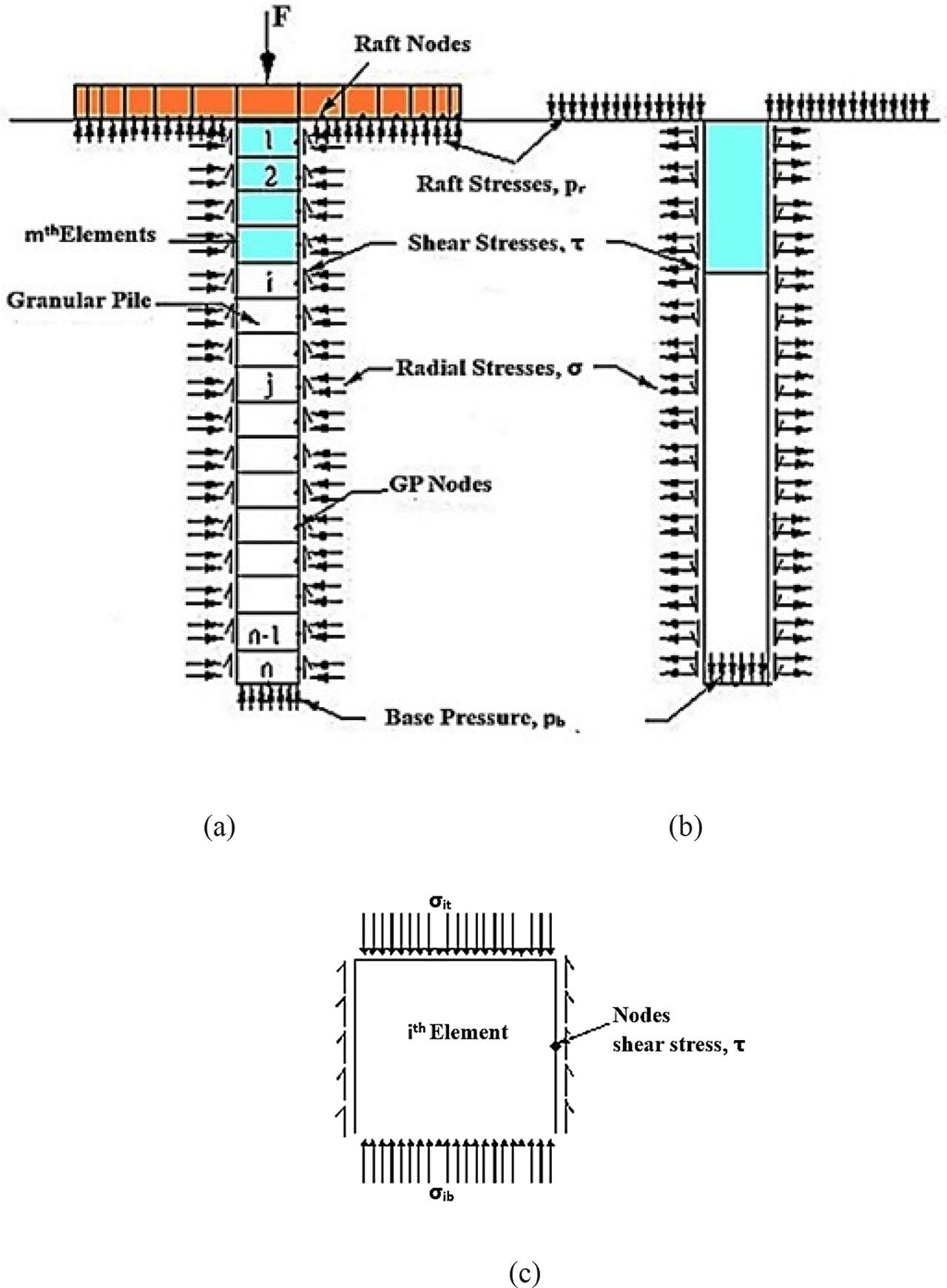(a) Stresses on the GP and raft due to soil. (b) Stresses in the soil due to GP and raft. (c) Stresses on any ith element of the GP.
Methodology

Mindlin’s and Boussinesq’s solutions for a point load in the interior and on the surface of a semi-infinite elastic solid are adopted to calculate the displacement of soil at the interface of the soil and the pile and the soil and the raft, respectively. Following are the assumptions made in the analysis:

– The soil ishomogeneous, isotropic, and linearly elastic.

– The sides of GP are perfectly rough with no slip.

– GP base is supposed to be smooth and rigid.

– Stress-strain relationships of soil and GP material are linear.

Soil Displacement

Displacements along GP–soil interface are evaluated at the midpoint on the side of each element and at the center of the base by integration of Mindlin’s and Boussinesq’s expressions based on the influence of the elemental stresses of GP and the raft stresses, respectively, in matrix form following Sharma and Madhav . ${ρpv}={Spvdp}=[ivv]{τEs}+[ivra]{σrEs}+[ivr]{prEs}$ \left\{{{\rho ^{pv}}} \right\} = \left\{{{{{S^{pv}}} \over {{d_p}}}} \right\} = \left[ {{i^{{\rm{vv}}}}} \right]\left\{{{\tau \over {{E_s}}}} \right\} + \left[ {{i^{vra}}} \right]\left\{{{{{\sigma _r}} \over {{E_s}}}} \right\} + \left[ {{i^{{\rm{vr}}}}} \right]\left\{{{{{p_r}} \over {{E_s}}}} \right\} where {Spv} and {ρpv} are vertical and normalized vertical soil settlement (VSS) vectors (superscript letters stand for ‘p’ pile, ‘v’ vertical shear stress,‘ra’ radial stresses, and ‘r’ raft stresses), [ivv], [ivra], and [ivr] are matrix of size (n+1)×(n+1),(n+1)×n, and (n+1)×kr, respectively, of the settlement impact factor (SIF), and {τ}and {pr} are column vectors of sizes {n+1}and {kr}, respectively.

The radial displacements at midpoints of the elements and on the interface are ${ρpra}={Spradp}=[irav]{τEs}+[irara]{σrEs}+[irar]{prEs}$ \left\{{{\rho ^{{\rm{pra}}}}} \right\} = \left\{{{{{S^{pra}}} \over {{d_p}}}} \right\} = \left[ {{i^{rav}}} \right]\left\{{{\tau \over {{E_s}}}} \right\} + \left[ {{i^{rara}}} \right]\left\{{{{{\sigma _r}} \over {{E_s}}}} \right\} + \left[ {{i^{rar}}} \right]\left\{{{{{p_r}} \over {{E_s}}}} \right\} where {Spra} and {ρpra} are radial and normalized VSS vectors, and [irav], [irara], and [irar] are matrix of size n×(n+1), n×n, and n×kr, respectively, of the SIF.

Displacements for soil–raft nodes are evaluated based on the interaction of elemental stresses from raft and GP. Soil displacement equations for raft nodes in matrix form are ${ρr}={Srdp}=[irv]{τEs}+[irra]{σrEs}+[irr]{prEs}$ \left\{{{\rho ^r}} \right\} = \left\{{{{{S^r}} \over {{d_p}}}} \right\} = \left[ {{i^{rv}}} \right]\left\{{{\tau \over {{E_s}}}} \right\} + \left[ {{i^{rra}}} \right]\left\{{{{{\sigma _r}} \over {{E_s}}}} \right\} + \left[ {{i^{rr}}} \right]\left\{{{{{p_r}} \over {{E_s}}}} \right\} where {Sr} and {ρr} are vertical and normalized VSS vector, and [irv], [irra], and [irr] are matrix of size kr×(n+1), kr×n, and kr×kr, respectively, of the SIF.

GP Displacement

Generalized stress-strain relation is utilized to evaluate the vertical and radial displacements of the components or elements of the GP as $εv=[σv−2vpσrr]Epandεθ=εr=[σr−vp(σr+σv)]Ep$ \matrix{{{\varepsilon _v} = {{\left[ {{\sigma _v} - 2{v_p}{\sigma _{rr}}} \right]} \over {{E_p}}}{\rm{and}}} \cr {{\varepsilon _\theta} = {\varepsilon _r} = {{\left[ {{\sigma _r} - {v_p}\left({{\sigma _r} + {\sigma _v}} \right)} \right]} \over {{E_p}}}} \cr} where εv, εq, and εr are, respectively, the axial, tangential, and radial strains of an element. σv and σr are the axial and radial stresses on the element, respectively.

Relationship Between Axial and Shear Stresses of GP

The axial load, F, applied at the top of the GP is resisted by the shear stresses, τ, along the pile and the base pressure, pb, as $F=∑j=1j=(n)τjπdpLpn+pbπdp24$ F = \sum\nolimits_{j = 1}^{j = \left(n \right)} {{{{\tau _j}\pi {d_p}{L_p}} \over n}} + pb{{\pi d_p^2} \over 4} where ‘n’ is the number of components of GP.

The direct stress on an element ‘i’ is calculated by taking the average of the stresses on top of element σit and bottom of element σib, $σvi=σit+σib2=pb+∑j=(i+1)j=n4(Lp/dp)τjn+2(Lp/dp)τin$ {\sigma _{vi}} = {{{\sigma _{it}} + {\sigma _{ib}}} \over 2} = {p_b} + \sum\nolimits_{j = \left({i + 1} \right)}^{j = n} {{{4\left({{L_p}/{d_p}} \right){\tau _j}} \over n} + {{2\left({{L_p}/{d_p}} \right){\tau _i}} \over n}}

The above equation relates the shear stresses to axial stresses along the surface of component and is deduced in matrix form as ${σv}=[A]{τ}$ \left\{{{\sigma _v}} \right\} = \left[ A \right]\left\{\tau \right\} where {τ} and {σv} are columns vectors of shear stresses of size (n+1). Matrix [A] represents the relation between axial and shear stresses in an upper triangular square matrix of size (n+1) for each element along the pile length and at the base of pile. $[A]=[2(Lp/dp)n4(Lp/dp)n4(Lp/dp)n4(Lp/dp)n––––102(Lp/dp)n4(Lp/dp)n4(Lp/dp)n––––1002(Lp/dp)n4(Lp/dp)n––––1––––––––––––––––––––––––––––––––02(Lp/dp)n4(Lp/dp)n1––––––02(Lp/dp)n1––––––001]$ \left[ A \right] = \left[ {\matrix{{{{2\left({{L_p}/{d_p}} \right)} \over n}} & {{{4\left({{L_p}/{d_p}} \right)} \over n}} & {{{4\left({{L_p}/{d_p}} \right)} \over n}} & {{{4\left({{L_p}/{d_p}} \right)} \over n}} & {\rm{-}} & {\rm{-}} & {\rm{-}} & {\rm{-}} & 1 \cr 0 & {{{2\left({{L_p}/{d_p}} \right)} \over n}} & {{{4\left({{L_p}/{d_p}} \right)} \over n}} & {{{4\left({{L_p}/{d_p}} \right)} \over n}} & {\rm{-}} & {\rm{-}} & {\rm{-}} & {\rm{-}} & 1 \cr 0 & 0 & {{{2\left({{L_p}/{d_p}} \right)} \over n}} & {{{4\left({{L_p}/{d_p}} \right)} \over n}} & {\rm{-}} & {\rm{-}} & {\rm{-}} & {\rm{-}} & 1 \cr {\rm{-}} & {\rm{-}} & {\rm{-}} & {\rm{-}} & {\rm{-}} & {\rm{-}} & {\rm{-}} & {\rm{-}} & {\rm{-}} \cr {\rm{-}} & {\rm{-}} & {\rm{-}} & {\rm{-}} & {\rm{-}} & {\rm{-}} & {\rm{-}} & {\rm{-}} & {\rm{-}} \cr {\rm{-}} & {\rm{-}} & {\rm{-}} & {\rm{-}} & {\rm{-}} & {\rm{-}} & {\rm{-}} & {\rm{-}} & {\rm{-}} \cr {\rm{-}} & {\rm{-}} & {\rm{-}} & {\rm{-}} & {\rm{-}} & 0 & {{{2\left({{L_p}/{d_p}} \right)} \over n}} & {{{4\left({{L_p}/{d_p}} \right)} \over n}} & 1 \cr {\rm{-}} & {\rm{-}} & {\rm{-}} & {\rm{-}} & {\rm{-}} & {\rm{-}} & 0 & {{{2\left({{L_p}/{d_p}} \right)} \over n}} & 1 \cr {\rm{-}} & {\rm{-}} & {\rm{-}} & {\rm{-}} & {\rm{-}} & {\rm{-}} & 0 & 0 & 1 \cr}} \right]

The vertical settlements of pile nodes on each component are calculated from the settlements of top of GP, ρt, to bottom component by considering the strain of each element successively. The settlement, ρip, of any element ‘i’ is obtained as $ρip=ρt−∑j=1j=(i−1) εvjΔzdp−εviΔz2dp$ \rho _i^p = {\rho _t} - \sum\nolimits_{j = 1}^{j = \left({i - 1} \right)} {\,{\varepsilon _{vj}}} {{\Delta z} \over {{d_p}}} - {\varepsilon _{vi}}{{\Delta z} \over {2{d_p}}} where εvi and εvj are the axial strains of ith and jth elements, respectively.

To calculate the settlement of the base of GP, the strain at the base is $εb=−dSpdz=pbEb$ {\varepsilon _b} = - {{d{S^p}} \over {dz}} = {{{p_b}} \over {{E_b}}}

Using finite difference scheme with unequal intervals of spacing, the above equation is $4Sn−1p−36Snp+32Sn+1p12(Δz/dp)=−pbEp$ {{4S_{n - 1}^p - 36S_n^p + 32S_{n + 1}^p} \over {12\left({\Delta z/{d_p}} \right)}} = - {{{p_b}} \over {{E_p}}} where $Sn−1p$ S_{n - 1}^p , $Snp$ S_n^p , and $Sn+1p$ S_{n + 1}^p are the settlements of elements n − 1, n, and n+1, respectively. Rewriting equation (18) in the normalized form, one gets $4ρn−1p−36ρnp+32ρn+1p=−pbEp12(Lp/dp)n$ 4\rho _{n - 1}^p - 36\rho _n^p + 32\rho _{n + 1}^p = - {{{p_b}} \over {{E_p}}}{{12\left({{L_p}/{d_p}} \right)} \over n}

By putting the values of $ρn−1p$ \rho _{n - 1}^p and $ρnp$ \rho _n^p from Eq. (9), and rearranging the terms, one gets $ρn+1p=ρt−∑j=1j=mεvjΔzdp−∑j=m+1j=(n−2)εviΔzdp− −3432εv(n−1)Δzdp−1832εvnΔzdp−632(L/d)nKppbES$ \matrix{{\rho _{n + 1}^p = {\rho _t} - \sum\nolimits_{j = 1}^{j = m} {{\varepsilon _{vj}}} {{\Delta z} \over {{d_p}}} - \sum\nolimits_{j = m + 1}^{j = \left({n - 2} \right)} {{\varepsilon _{{\rm{vi}}}}} {{\Delta z} \over {{d_p}}} -} \hfill \cr {\,\,\,\,\, - {{34} \over {32}}{\varepsilon _{v\left({n - 1} \right)}}{{\Delta z} \over {{d_p}}} - {{18} \over {32}}{\varepsilon _{vn}}{{\Delta z} \over {{d_p}}} - {6 \over {32}}{{\left({L/d} \right)} \over {n{K_p}}}{{{p_b}} \over {{E_S}}}} \hfill \cr}

In the above equation, for the calculation of εvj, Kstp = Estp/Es comparative strengthening of strengthened portion with soil is taken, while for calculation of εvi, and other εvn, and εv(n-1), Kp = Ep/Es comparative strengthening of unstrengthened portion with soil is considered.

Combining Eqs (9) and (13), the vertical settlements of GP are ${ρppv}=ρt{1}+[B]{σvES}+[C]{σrES}$ \left\{{{\rho ^{ppv}}} \right\} = {\rho _t}\left\{1 \right\} + \left[ B \right]\left\{{{{{\sigma _v}} \over {{E_S}}}} \right\} + \left[ C \right]\left\{{{{{\sigma _r}} \over {{E_S}}}} \right\} where [B] and [C] are lower triangular matrices of sizes (n+1)×(n+1) and(n+1) × (n), which are given by $[B]=(Lp/dp)nKp[−0.5μr000––––0−1μr−0.5μr00––––0−1μr−1μr−0.5μr0–––––––––––––––––––––––––––––––––––––––––−1−1–––––−0.50−1−1––––−3432−1832−632][C]=vp(Lp/dp)nKp[1μr000––––2μr1μr00––––2μr2μr1μr0––––––––––––––––––––––––––––––––––––22–––––122–––683236320]$ \matrix{{\left[ B \right] = {{\left({{L_p}/{d_p}} \right)} \over {n{K_p}}}\left[ {\matrix{{{{- 0.5} \over {{\mu ^r}}}} & 0 & 0 & 0 & {\rm{-}} & {\rm{-}} & {\rm{-}} & {\rm{-}} & 0 \cr {{{- 1} \over {{\mu ^r}}}} & {{{- 0.5} \over {{\mu ^r}}}} & 0 & 0 & {\rm{-}} & {\rm{-}} & {\rm{-}} & {\rm{-}} & 0 \cr {{{- 1} \over {{\mu ^r}}}} & {{{- 1} \over {{\mu ^r}}}} & {{{- 0.5} \over {{\mu ^r}}}} & 0 & {\rm{-}} & {\rm{-}} & {\rm{-}} & {\rm{-}} & {\rm{-}} \cr {\rm{-}} & {\rm{-}} & {\rm{-}} & {\rm{-}} & {\rm{-}} & {\rm{-}} & {\rm{-}} & {\rm{-}} & {\rm{-}} \cr {\rm{-}} & {\rm{-}} & {\rm{-}} & {\rm{-}} & {\rm{-}} & {\rm{-}} & {\rm{-}} & {\rm{-}} & {\rm{-}} \cr {\rm{-}} & {\rm{-}} & {\rm{-}} & {\rm{-}} & {\rm{-}} & {\rm{-}} & {\rm{-}} & {\rm{-}} & {\rm{-}} \cr {\rm{-}} & {\rm{-}} & {\rm{-}} & {\rm{-}} & {\rm{-}} & {\rm{-}} & {\rm{-}} & {\rm{-}} & {\rm{-}} \cr {- 1} & {- 1} & {\rm{-}} & {\rm{-}} & {\rm{-}} & {\rm{-}} & {\rm{-}} & {- 0.5} & 0 \cr {- 1} & {- 1} & {\rm{-}} & {\rm{-}} & {\rm{-}} & {\rm{-}} & {- {{34} \over {32}}} & {- {{18} \over {32}}} & {- {6 \over {32}}} \cr}} \right]} \cr {\left[ C \right] = {{{v_p}\left({{L_p}/{d_p}} \right)} \over {n{K_p}}}\left[ {\matrix{{{1 \over {{\mu ^r}}}} & 0 & 0 & 0 & {\rm{-}} & {\rm{-}} & {\rm{-}} & {\rm{-}} \cr {{2 \over {{\mu ^r}}}} & {{1 \over {{\mu ^r}}}} & 0 & 0 & {\rm{-}} & {\rm{-}} & {\rm{-}} & {\rm{-}} \cr {{2 \over {{\mu ^r}}}} & {{2 \over {{\mu ^r}}}} & {{1 \over {{\mu ^r}}}} & 0 & {\rm{-}} & {\rm{-}} & {\rm{-}} & {\rm{-}} \cr {\rm{-}} & {\rm{-}} & {\rm{-}} & {\rm{-}} & {\rm{-}} & {\rm{-}} & {\rm{-}} & {\rm{-}} \cr {\rm{-}} & {\rm{-}} & {\rm{-}} & {\rm{-}} & {\rm{-}} & {\rm{-}} & {\rm{-}} & {\rm{-}} \cr {\rm{-}} & {\rm{-}} & {\rm{-}} & {\rm{-}} & {\rm{-}} & {\rm{-}} & {\rm{-}} & {\rm{-}} \cr {\rm{-}} & {\rm{-}} & {\rm{-}} & {\rm{-}} & {\rm{-}} & {\rm{-}} & {\rm{-}} & {\rm{-}} \cr 2 & 2 & {\rm{-}} & {\rm{-}} & {\rm{-}} & {\rm{-}} & {\rm{-}} & 1 \cr 2 & 2 & {\rm{-}} & {\rm{-}} & {\rm{-}} & {{{68} \over {32}}} & {{{36} \over {32}}} & 0 \cr}} \right]} \cr} Here in matrix [B] and [C], Kstp will be replaced by μr× Kp for top elements of GP to take into consideration of the effect of strengthening upto a depth λrLp/dp.

Using the shear and axial stresses relationship (Eq. 7), the vertical settlement of GP nodes in terms of shear stresses and radial stresses is ${ρppv}=ρt{1}+[D]{τES}+[C]{σrES}$ \left\{{{\rho ^{ppv}}} \right\} = {\rho _t}\left\{1 \right\} + \left[ D \right]\left\{{{\tau \over {{E_S}}}} \right\} + [C]\left\{{{{{\sigma _r}} \over {{E_S}}}} \right\} where [D] is a square matrix of size, (n+1) = [B] [A].

The radial displacements of nodes are calculated depending on stress-strain relationship for the axisymmetric case as $εθ=Sppra=−[(1−vp)σr−vpσv]Ep$ {\varepsilon _\theta} = {{{S^{ppr}}} \over a} = - {{\left[ {\left({1 - {v_p}} \right){\sigma _r} - {v_p}{\sigma _v}} \right]} \over {{E_p}}} where εq, Sppr, and a are the tangential strain, radial displacement, and radius of GP, respectively. The equation in normalized and matrix form is ${ρppr}={Spprdp}=[E]{σvES}+[F]{σrES}$ \left\{{{\rho ^{ppr}}} \right\} = \left\{{{{{S^{ppr}}} \over {{d_p}}}} \right\} = \left[ E \right]\left\{{{{{\sigma _v}} \over {{E_S}}}} \right\} + \left[ F \right]\left\{{{{{\sigma _r}} \over {{E_S}}}} \right\} where [E] and [F] are the matrices of sizes n×(n+1) and n×n, respectively, and are $[E]=vp2Kp[1μr0––001μr––––––––––––––––10][F]=−(1−vp)2Kp[U]$ \matrix{{\left[ E \right] = {{{v_p}} \over {2{K_p}}}\left[ {\matrix{{{1 \over {{\mu ^r}}}} & 0 & - & - & 0 \cr 0 & {{1 \over {{\mu ^r}}}} & - & - & - \cr - & - & - & - & - \cr - & - & - & - & - \cr - & - & - & 1 & 0 \cr}} \right]} \cr {\left[ F \right] = - {{\left({1 - {v_p}} \right)} \over {2{K_p}}}\left[ U \right]} \cr} where [U] is a unit matrix of size ‘n’. The radial displacements of GP in terms of interfacial shear stresses of GP and soil (Eqs (7) and (18)) are ${ρppr}=[G]{τES}+[F]{σrES}$ \left\{{{\rho ^{ppr}}} \right\} = \left[ G \right]\left\{{{\tau \over {{E_S}}}} \right\} + \left[ F \right]\left\{{{{{\sigma _r}} \over {{E_S}}}} \right\} where [G] is a matrix of size n×(n+1) = [E][A].

Raft Settlements

Raft is considered as rigid and hence settlements of raft nodes are all equal. The settlement of the top of the GP (ρt) is equal to raft settlement and expressed as ${ρpr}=ρt{1}$ \left\{{{\rho ^{pr}}} \right\} = {\rho _t}\left\{1 \right\} where {ρpr} is the raft displacement vector of size ‘kr’.

Compatibility of Settlements

Satisfying the compatibility of settlements for GP, raft, and soil, solutions are obtained in terms of shear and radial and raft stresses at the GP–soil and raft–soil interfaces, respectively. Applying the compatibility condition for vertical settlements of GP (Eqs (1) and (16)) ${ρpv}={ρppv} or[AA]{τES}+[BB]{σrES}+[ivr]{prES}=ρt{1}$ \matrix{{\left\{{{\rho ^{pv}}} \right\} = \left\{{{\rho ^{ppv}}} \right\}\,{\rm{or}}} \cr {\left[ {AA} \right]\left\{{{\tau \over {{E_S}}}} \right\} + \left[ {BB} \right]\left\{{{{{\sigma _r}} \over {{E_S}}}} \right\} + \left[ {{i^{vr}}} \right]\left\{{{{{p_r}} \over {{E_S}}}} \right\} = {\rho _t}\left\{1 \right\}} \cr} where [AA]=[ivv]−[D] of size (n+1)×(n+1) and [BB]=[ivra]−[C] of size (n+1)×n.

Satisfying compatibility of radial displacements of GP, i.e., equating Eqs (2) and (20) $[CC]{τES}+[DD]{σrES}+[irar]{prES}={0}$ \left[ {CC} \right]\left\{{{\tau \over {{E_S}}}} \right\} + \left[ {DD} \right]\left\{{{{{\sigma _r}} \over {{E_S}}}} \right\} + \left[ {{i^{rar}}} \right]\left\{{{{{p_r}} \over {{E_S}}}} \right\} = \left\{0 \right\} where [CC]=[irav]-[G] is of size n×(n+1) and [DD]=[irara]-[F] is of size n×n.

For the compatibility of displacements of the raft (Eqs (3) and (21)) ${ρr}={ρpr}or[irv]{τES}+[irra]{σrES}+[irr]{prES}=ρt{1}$ \matrix{{\left\{{{\rho ^r}} \right\} = \left\{{{\rho ^{pr}}} \right\}{\rm{or}}} \cr {\left[ {{i^{rv}}} \right]\left\{{{\tau \over {{E_S}}}} \right\} + \left[ {{i^{rra}}} \right]\left\{{{{{\sigma _r}} \over {{E_S}}}} \right\} + \left[ {{i^{rr}}} \right]\left\{{{{{p_r}} \over {{E_S}}}} \right\} = {\rho _t}\left\{1 \right\}} \cr} Simultaneous equations (29), (30), and (31) are solved to obtain the interfacial shear and radial stresses along GP with raft stresses at raft–soil interface. Finally, the displacements of raft and GP nodes are obtained. $Sp=FESdpip$ {S^p} = {F \over {{E_S}{d_p}}}{i_p} where ip is SIF; under the axial load ‘F’ on partially strengthened granular piled raft, the settlement along the length of GP is calculated in terms of settlement influence factor (SIF), linked with other parameters μr, λr, and Kp.

Under the axial load ‘F’ on partially strengthened granular piled raft, the SIF for any depth, ipd, is also defined as $Spd=FESdpipd$ {S^{pd}} = {F \over {{E_S}{d_p}}}{i_{pd}} where ipd is SIF for any depth z of GP.

Similarly the radial displacement of GP at any depth can be expressed as $Ur=FESdpir$ {U_r} = {F \over {{E_S}{d_p}}}{i_r} where ir is radial displacement impact factor.

Results are evaluated and discussed in terms of following parameters to quantify the effect of strengthening the GP.

Parameter μr strengthening factor is defined as follows: $μr=Kstp/Kp$ {\mu ^{\rm{r}}} = {{\rm{K}}_{{\rm{stp}}}}/{{\rm{K}}_{\rm{p}}}

Parameter λr comparative length of strengthening is defined as follows: $λr=Ls/Lp$ {\lambda ^{\rm{r}}} = {{\rm{L}}_{\rm{s}}}/{{\rm{L}}_{\rm{p}}}

All the parameters defined above are for partially strengthened GP having μr>1. If μr =1, they can be obtained for unstrengthened GP. The overall response of partially strengthened floating granular piled raft foundation is evaluated in terms of the vertical and radial displacement impact factors, the SIF for any depth, the normalized GP–soil interface shear and radial stresses, the load ratio, i.e., the percentage of the load taken by the GP to the total load, and the normalized contact pressure distribution below the raft. The parameters affecting the overall responses are (i) the geometric ones – the ratio of diameter of the raft to that of GP, i.e., diameter ratio, (dr/dp), and the length to diameter ratio of GP, (Lp/dp); (ii) comparative GP–soil stiffness, i.e., Kp = (Ep/Es); (iii) Poisson’s ratio of the soil, νs; (iv) strengthening factor μr = Kstp/Kp where Kstp = (Estp/Ep) and Kp = (Ep/Es); and (v) comparative length of strengthening of GP, λr.

Results and Discussion

Results obtained in this analysis have been validated with those of Madhav et al. (2009) for rigid raft on a single compressible unstrengthened floating pile analyses with or without radial displacement compatibility for radial displacement. The agreement has been very close as shown in Fig. 9. Results are obtained for the following ranges of non dimensional parameters, comparative length of GP, Lp/dp = 10–30, comparative strengthening, Kp of GP = 10–1000, comparative size of raft, dr/dp = 2–5, stiffness factor μr =1–10, and comparative length of strengthening from top of GP, λr = 0.1–0.4. Effect of Poisson’s ratios of surrounding soil and GP does not affect the results significantly. Although the realistic normal range of Kp for GP are 10–100, results are obtained for Kp= 1000.

In order to compare the values of SIFs, ip, obtained with and without satisfying radial displacement compatibility of nodes along GP, the variation of ip with comparative GP–soil stiffness, with Kp, is shown in Fig. 3. For dr/dp=3, Lp/dp=10, λr=0.4, and μr = 1, 2, 5, and 10, the value of SIF without radial compatibility and with radial compatibility are 0.227, 0.211, 0.194, and 0.185 and 0.224, 0.210, 0.193, and 0.184, respectively. The differences in the values of ip obtained from the two analyses are in the range of 1–1.5% and decrease with the increase of strengthening factor μr. Thus, the consideration of radial displacement compatibility of GP in the analysis does not influence the SIF significantly.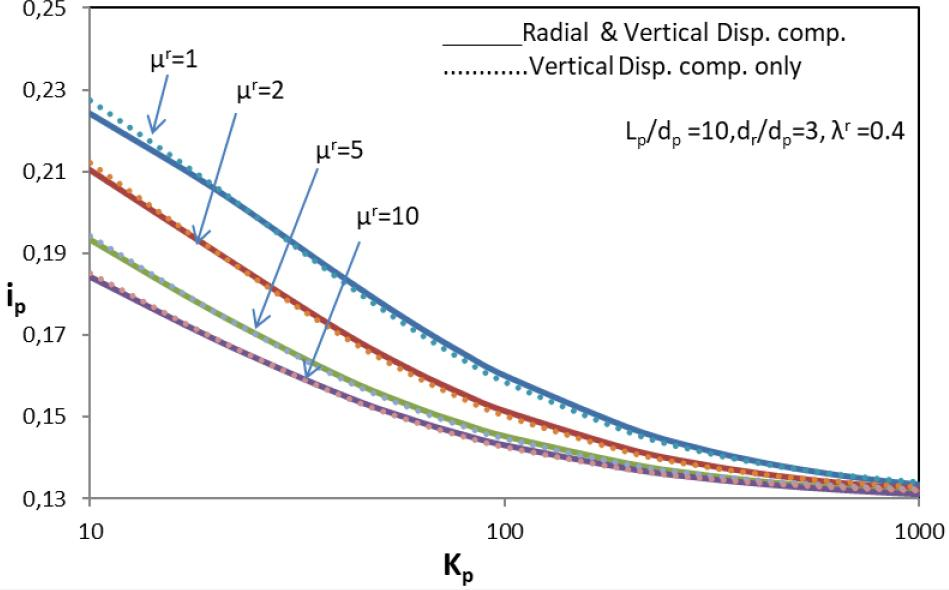Variation of SIF, ip, with comparative strengthening, Kp, of GP– effect of strengthening factor, μr, and radial and vertical settlement compatibility on partially strengthened GP–raft foundation (Lp/dp =10, dr/dp =3, λr =0.4).

Fig. 4 shows the effect of considering radial compatibility of displacement, on the value of SIF for top, ip, it is quite clear from Fig. 3 that as by considering the radial compatibility, increases, SIF for top, ip, decreases for all the values of comparative strengthening of GP, Kp. The increase in SIF is not significant because the percentage increase is only 1–2% at μr=1. The difference in the value of SIF is reducing with the increase in stiffness factor, μ, and comparative strengthening factor of GP, Kp. It can be seen as an illustration that for a partially strengthened granular piled raft, with Lp/dp=10, dr/dp=3 with λr=0.4 and Kp=10, 50, 100, and 1000 with radial and without radial compatibility are 0.224, 0.178, 0.160, and 0.133 and 0.227, 0.176, 0.158, and 0.134, respectively. The effect of stiffness factor, μr, can also be seen that as it increases, the SIF for top, ip, decreases because strengthening effect is enhancing.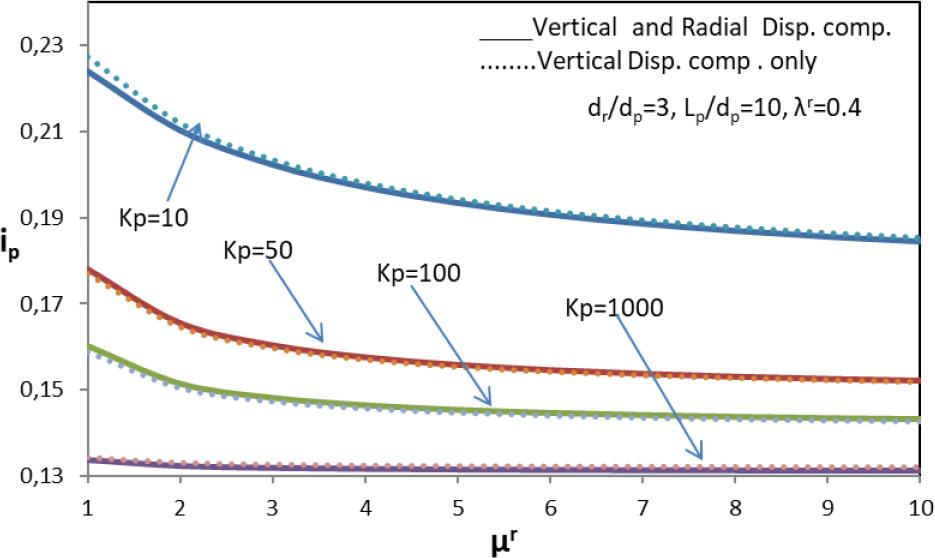Variation of SIF, ip, with comparative strengthening, μr, of GP– effect of comparative length of strengthening, Kp, of GP and radial and vertical settlement compatibility on partially strengthened GP–raft foundation (Lp/dp =10, dr/dp=3, λr =4).

Fig. 5 shows the effect of comparative length of strengthening, λr, from top of GP on SIF for top, ip, for comparative size of raft, dr/dp=3 and 5 with considering the radial compatibility of displacement. With the increase in length of strengthening from top of GP, the SIF decreases. For example, a look at the graph reveals that for a partially strengthened granular piled raft with Lp/dp=10, Kp=10, dr/dp=3, and λr= 0.2, 0.3, and 0.4, the values of ip are 0.210, 0.202, and 0.197, respectively, hence causing a percentage decrease in value of about 3.8 and 6.1, respectively, showing the aspects of percentage length of strengthening by reducing the SIF for top, ip.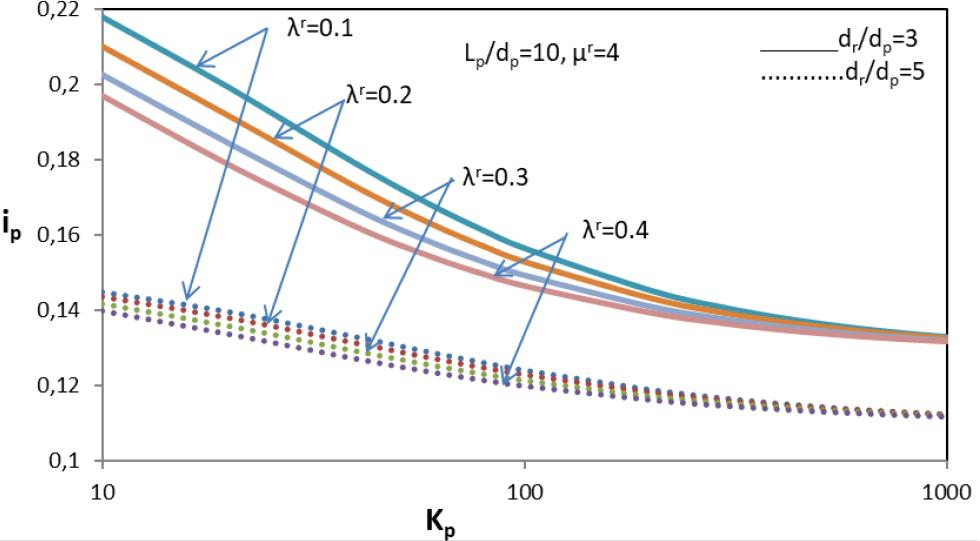Variation of SIF, ip, considering radial displacement with comparative strengthening, Kp, of GP– effect of comparative length, λr, of strengthening from top of GP and comparative size of raft, dr/dp, on partially strengthened GP–raft foundation (Lp/dp =10, μr=4).

Fig. 6 presents the variations of top SIF with the comparative strengthening of GP for Lp/dp=10, with comparative length of strengthening from top of GP, λr=0.4, stiffness factor, μr=1, 5, and 10, and comparative size of raft, dr/dp=3 and 5. As expected, the SIF, ip, decreases with the increase in the value of comparative strengthening, Kp, of GP. The SIF for single GP decreases from about 0.224 for homogeneous or unstrengthened GP (μ=1) at Kp= 10, and dr/dp=3, to about 0.193 and 0.184 for μr equal to 5 and 10, respectively. The percentage reduction in the SIF, ip, from μr=1 to 5 is 13.8 and from μr=1 to 10 is 17.8. It is also observed that as stiffness factor, μr, increases, the value of SIF, ip, decreases showing the effect of strengthening. This occurs due to the stiffer portion of GP transferring the stresses toward the lower portion and the base of the GP. The rate of decrease of SIF, Ip, reduces with the increase in comparative size of raft from dr/dp = 3–5.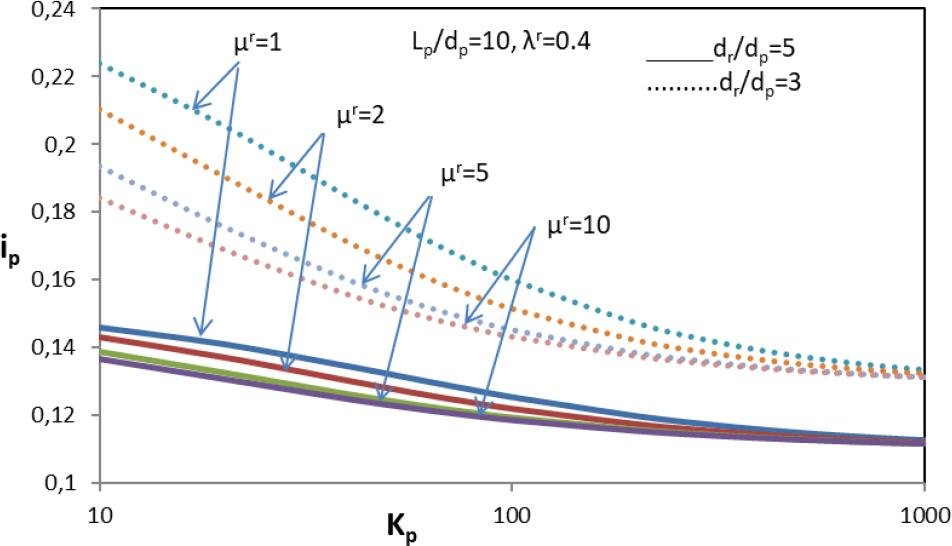Variation of SIF, ip, considering radial displacement with comparative strengthening, Kp, of GP– effect of strengthening factor, μr, and comparative size of raft, dr/dp, on partially strengthened GP–raft foundation (Lp/dp =10, λr =0.4).

Fig. 7 shows the variation of SIF, ip, with comparative strengthening, Kp, of GP with the effect of strengthening factor, μr, for Lp/dp=10, dr/dp=3, and μr= 1 and 5. The SIF, ip, decreases with increase in the strengthening factor, μr. The value of ip at Lp/dp =10, Kp=10, λr = 0.4, and dr/dp=3 is about 0.224 for μ=1 and the corresponding value for μr=5 is 0.193 with percentage decreases of 13.8%. It is also noticed that SIF decreases with the increase of comparative strengthening of GP, Kp.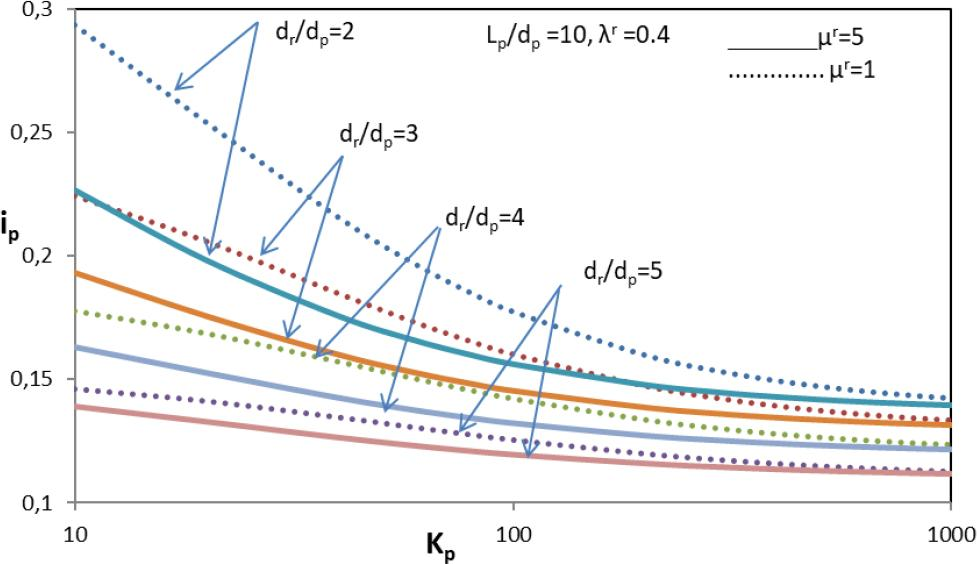Variation of SIF, ip, considering radial displacement with comparative strengthening, Kp, of GP– effect of comparative size of raft, dr/dp, and stiffness factor, μr, on partially strengthened GP–raft foundation (Lp/dp =10, λr =0.4).

The influence of comparative length, Lp/dp, of GP on the variation of SIF, ip, with comparative strengthening of GP, Kp, is shown in Fig. 8 for μr = 1 and 5. The SIF decreases with the increases of stiffness factor, μr. For Lp/dp= 10 and Kp = 10, ip decreases from 0.224 to 0.193 for μr increasing from 1 to 5. The values of ip for Kp = 10, μr=5, and Lp/dp=10, 20, and 30 are 0.193, 0.175, and 0.168, respectively. The percentage decreases in SIF, ip, are 9.3 and 12.9 for increases in Lp/dp from 10 to 20 and from 20 to 30, respectively. The rate of decrease of SIF, ip, decreases with Kp and with increase in comparative length, Lp/dp.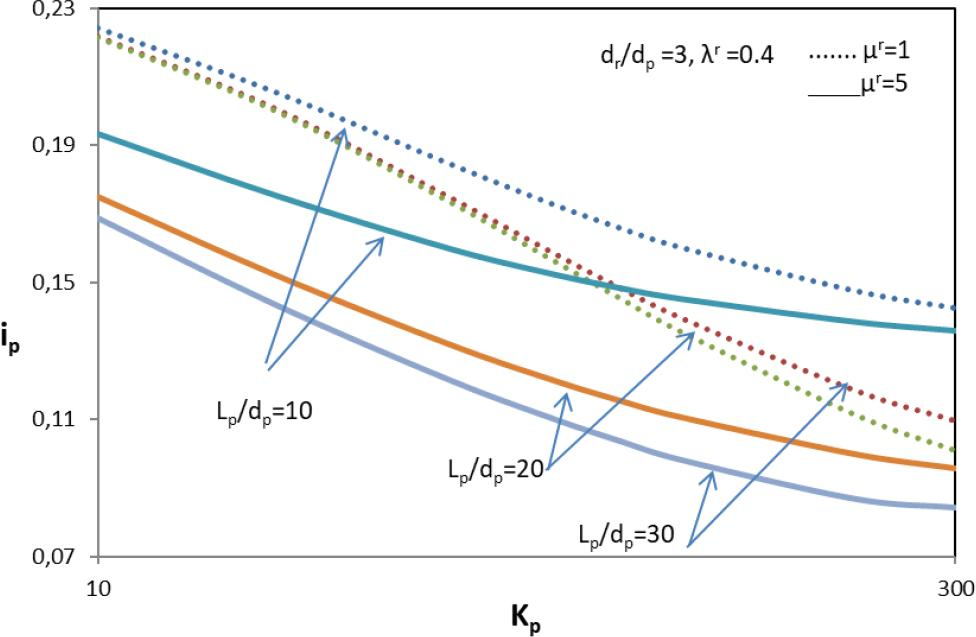Variation of SIF, ip, considering radial displacement with comparative strengthening, Kp, of GP– effect of comparative length of GP, Lp/dp, and stiffness factor, μr, on partially strengthened GP– raft foundation (dr/dp=3, λr =0.4).

Fig. 9 shows the influence of strengthening factor on radial displacement impact factor, ir, for dr/dp = 2, 3, and 5. The increase of strengthening factor, μr, from 1 to 5 increases the radial displacement impact factor, ir, and decreases along the depth of GP which shows the effect of strengthening in upper part. For strengthening factor, μr =1 (unstrengthened) GP, the results are validated with the Madhav et al.  and found the variation trends are same. With the increase in comparative size of raft, dr/dp, the radial displacement impact factor, ir, decreases at the top. The influence of normal stresses from raft on radial displacements of GP depends on their magnitude and comparative distances from the GP. Therefore, the maximum radial displacements of GP decrease and shift downward with the increase of dr/dp due to increase in load carried by raft, resulting in an increase in the confinement effect of raft. The radial displacement influence factor, ir, decreases with the increase of comparative size of raft, dr/dp, except in the lower region of GP where it increases as shown in Fig. 9.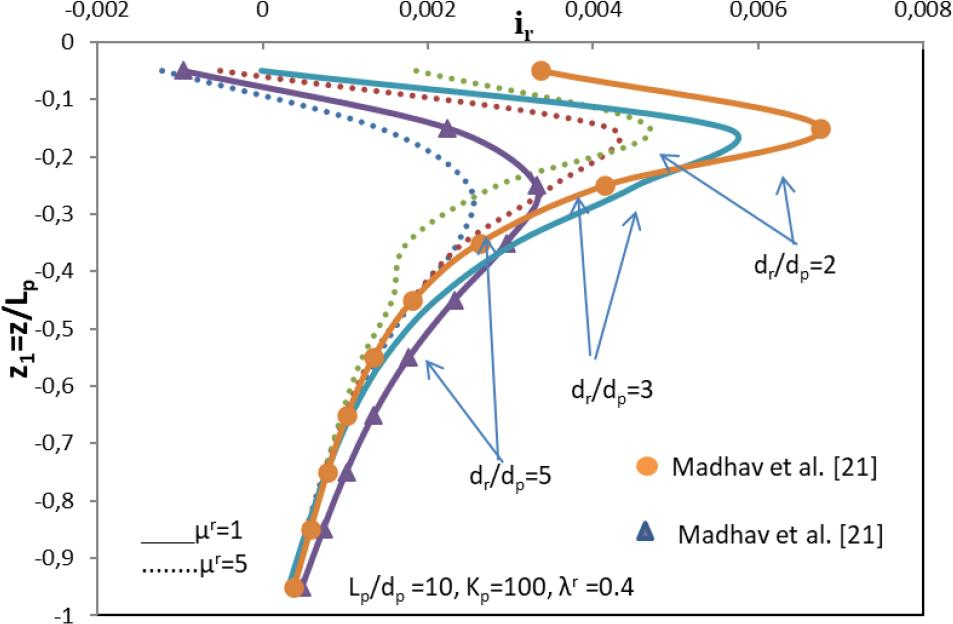Variation of radial displacement impact factor, ir, with comparative length of pile z1 = z/Lp – effect of strengthening factor μr and comparative size of raft, dr/dp, and radial and vertical settlement compatibility on partially strengthened GP–raft foundation (Lp/dp=10, Kp=100, λr =0.4).

The radial displacement influence factor, ir of GP, decreases along its depth with the increase of comparative strengthening of GP, Kp, as shown in Fig 10. This decrement in ir with Kp is more for relatively smaller value of strengthening factor (μr=1) as compared to the reduction in case of larger one (μr=5). The maximum value of ir for μr=1 is observed at a depth of 0.25L and its value decreases from 0.0033 for Kp = 50 to 0.0027 for μr=5. The percentage decrease in radial displacement impact factor, ip, is 18.18 for increase in μr from 1 to 5.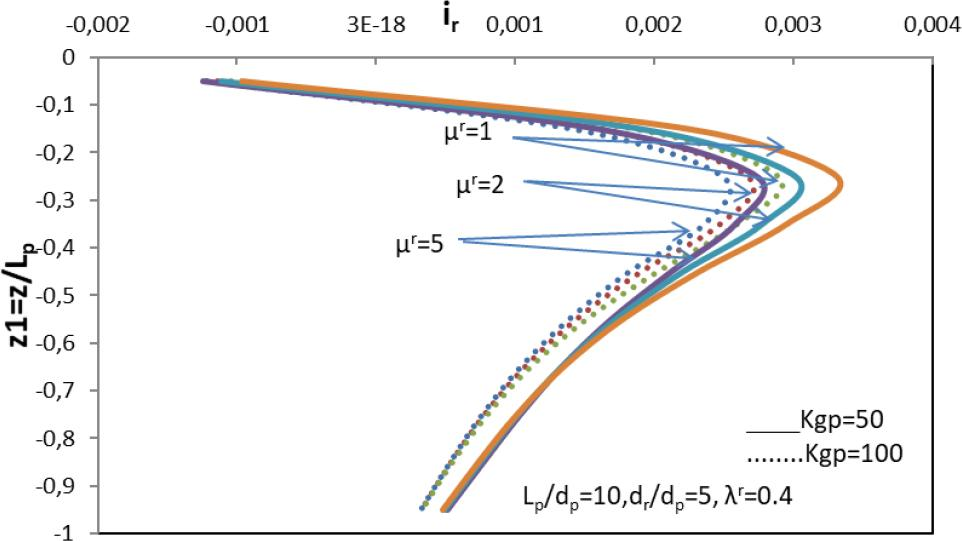Variation of radial SIF, ir, with the normalized depth, z1=z/Lp – effect of comparative strengthening, Kp, of GP and strengthening factor, μr, on a partially strengthened GP–raft foundation (Lp/dp=10, dr/dp=5, λr=0.4).

The variation of radial displacement influence factor, ir, with normalized depth, z1= (z/Lp), is shown in Fig. 11 for comparative length of strengthening, λr, of GP ranging from 0.2 to 0.4 and for Lp/dp = 10. The radial displacement influence factor, ir, increases at the top and decreases continuously with the depth of GP for the comparative length of strengthening, λr, in the range of 0.2–0.4. The values of radial displacement influence factor, ir, decrease from 0.0041 to 0.0035 at z1=0.25 for Lp/dp=10, dr/dp=3, μr=4, Kp=100 with the increases of λr=0.2–0.4, and the percentage decrease in radial displacement influence factor, ir, is around 17.14% which shows the effect of strengthening.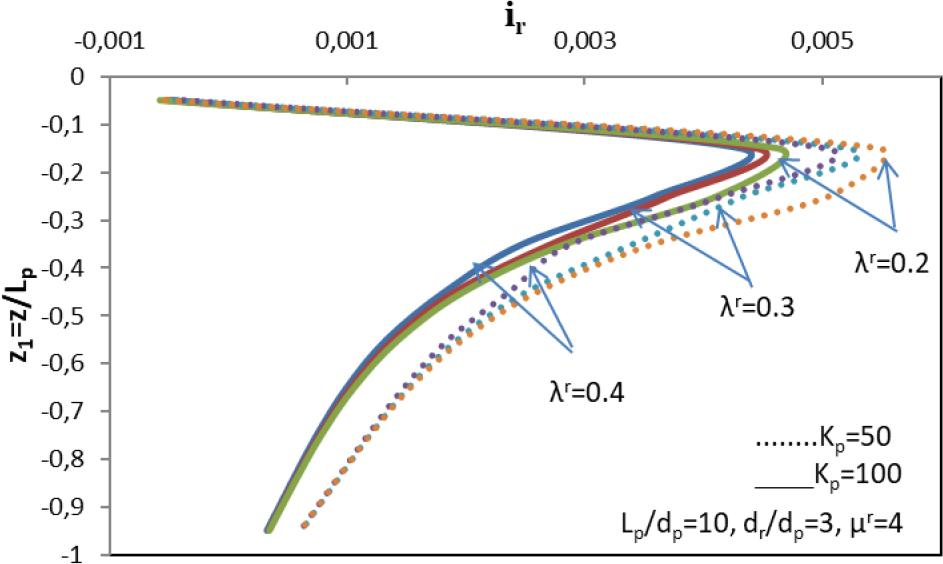Variation of radial SIF, ir, with the normalized depth, z1=z/Lp – effect of comparative strengthening, Kp, of GP and comparative length of strengthening, λr, on a partially strengthened GP–raft foundation (Lp/dp=10, dr/dp =3, μr=4).

Fig. 12 clearly shows that due to increases in the value of comparative strengthening of GP, Kp, overall the values of τ* decrease. The effect of strengthening factor, μr, can also be seen that as it increases, the shear stresses, τ*, increase because strengthening effect is enhancing. It may be seen as an example that for partially strengthened floating granular piled raft with Lp/dp=10, Kp=100, dr/dp=3, λr=0.4, and μr=1, 2, and 5, the value of τ* at z1=0.45 are 0.81, 1.02, and 1.16, respectively.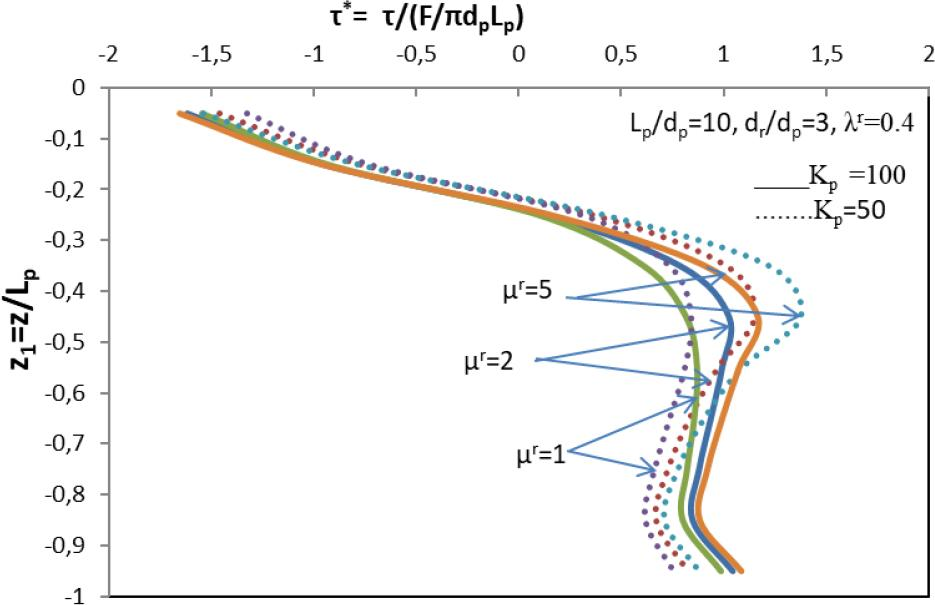Variation of normalized shear stresses, τ*= τ/(F/πdpLp), with radial compatibility of the normalized depth, z1 =z/Lp – effect of stiffness factor, μr, and comparative strengthening, Kp, of GP on a partially strengthened GP–raft foundation (Lp/dp=10, dr/dp=5, λr =4).

Fig. 13 clearly shows that as comparative length of strengthening, λr, increases, the normalized shear stresses increase. It is well noted that shear stresses are negative in upper part due to raft above the pile. The interfacial shear stresses upto length z1=0.25 are negative, while in lower portion, stress becomes positive and goes on increasing. For Lp/dp=10, μr=2, dr/dp=3, Kp=100, and λr=0.2, 0.3, and 0.4 at z1=0.45, the values of τ*= τ(πdpLp)/F are 0.85, 0.91, and 1.02, respectively, hence causing a percentage increase of 7 and 20. It can be seen as the shear stresses increase very steeply with the depth upto the comparative length of strengthening, λr=0.4, with the comparative strengthening of GP, Kp, these stresses are redistributed in lower portion of GP.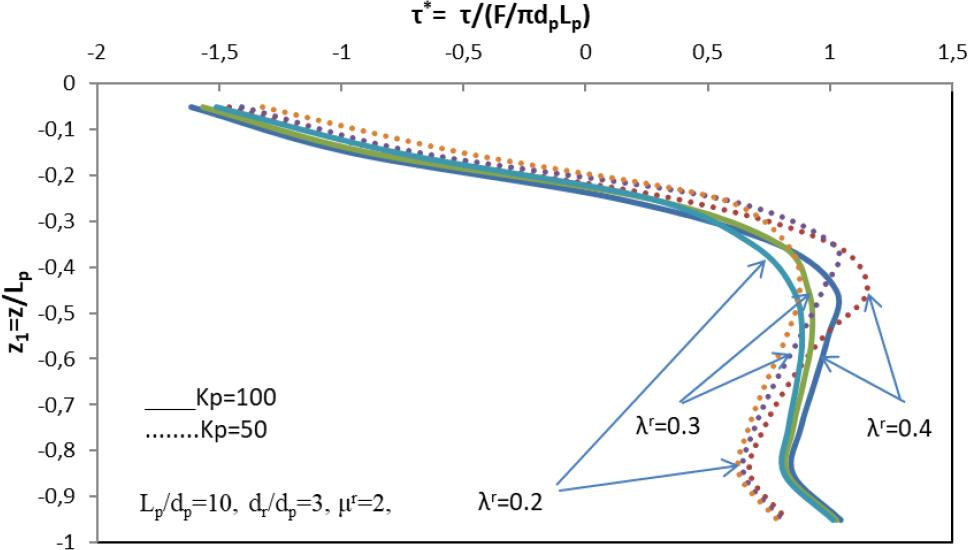Variation of normalized shear stresses, τ*= τ/(F/πdpLp), with radial compatibility of the normalized depth, z1=z/Lp – effect of stiffness factor, μ, and comparative strengthening, Kp, of GP on a partially strengthened GP–raft foundation (Lp/dp=10, dr/dp=3,μr=2).

The variations of radial stresses on GP, i.e., σr*(σr/(F/πdpLp)), with normalized depth of GP, z1= (z/Lp), are shown in Fig. 14, showing the effect of comparative size of raft, dr/dp, and comparative strengthening of GP, Kp. The increase of dr/dp from 3 to 5 the radial stresses along the depth of GP decreases upto the length of z1=0.55 after that radial stresses are increases due to normal stresses underneath the raft provide the confinement effect to GP decrease. With the increase of Kp, the radial stresses on GP increase along its depth. The effect of Kp on the radial stress distribution for comparative size of raft dr/dp = 3 is very similar to that obtained for dr/dp = 5 except that they decrease slightly with the increase of Kp in the top region of GP in the former case.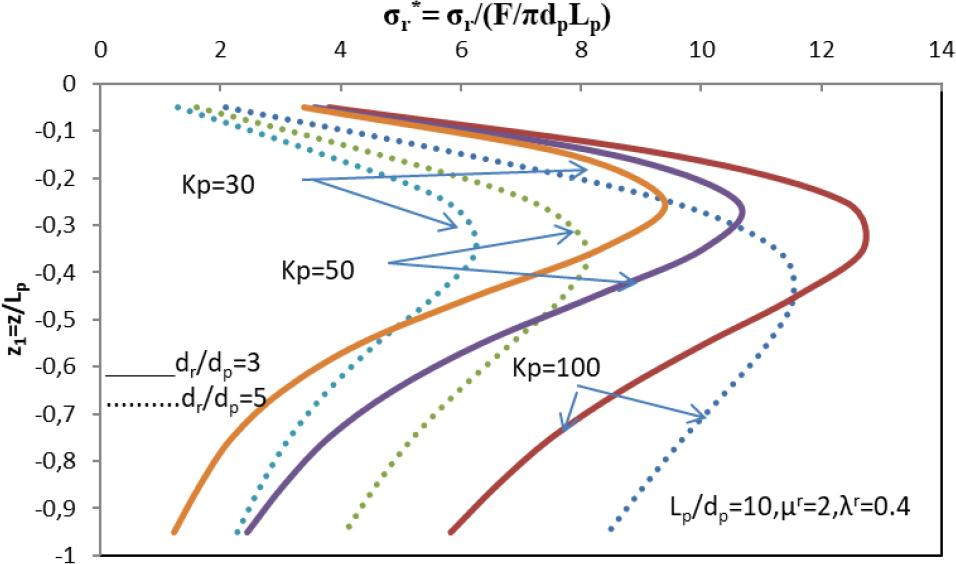Variation of normalized radial stresses, σr* = σr (πdpLp)/F, with the normalized depth, z1=z/Lp – effect of stiffness factor, μr, and comparative strengthening, Kp, of GP on a partially strengthened GP–raft foundation (Lp/dp=10, μr=2, λr=0.4).

The variations of radial stresses normalized with the total load on GP, i.e., σr*= σr (πdpLp)/F, with normalized depth of GP, z1= (z/Lp), are shown in Fig. 15, showing the influence of strengthening factor, μr. The radial stresses of GP increase upto the depth of strengthening in the range z1 = 0–4, and after that, they decrease with depth z1 > 4. With the increase in stiffness factor, μ, the radial stresses on GP increase along with its depth of strengthening and redistributed in lower part of pile. This result follows from the increase in the load carrying capacity of upper stiffer part is increased GP with the increase of GP stiffness and capacity of lower unstrengthened part remaining constant.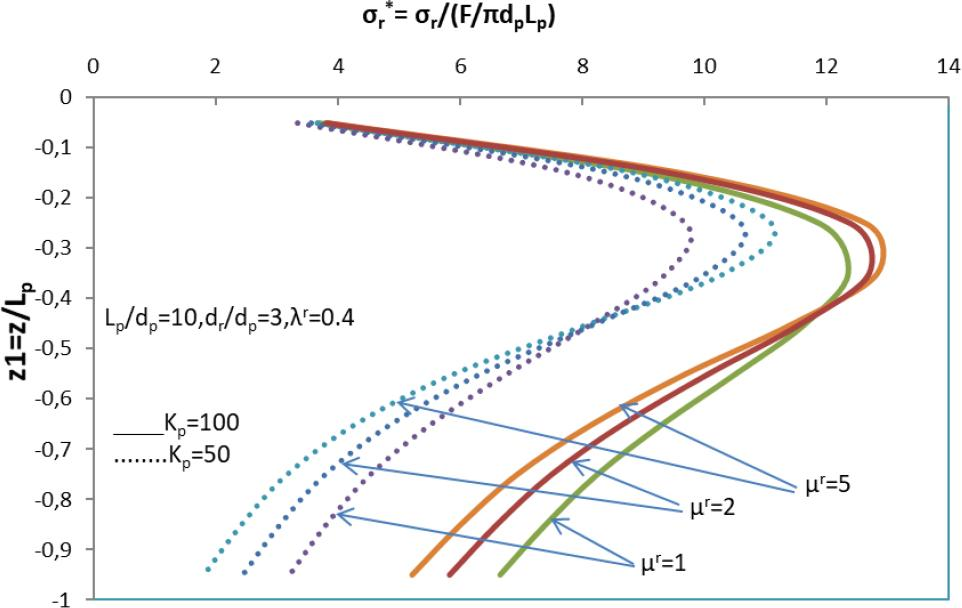Variation of normalized radial stresses, σr*= σr(πdpLp)/F, with the normalized depth, z1=z/Lp – effect of stiffness factor, μr, and comparative strengthening, Kp, of GP on a partially strengthened GP–raft foundation (Lp/dp=10, dr/dp=3, λr=0.4).

Fig. 16 shows the variation of SIF, ipd, of a GP with normalized depth, z1=z/Lp, for unstrengthened and strengthened GPs with raft for Lp/dp=10, Kp=100, dr/dp=3, and λr=0.4. Compatibility displacements at the interface of strengthened and unstrengthened portions of the GP are well satisfied. As strengthening factor, μr, changes from unstrengthened condition of GP, i.e., with μr =1 to strengthened condition with μr =2, 5, and 10, the SIFs, ipd, for top are, respectively, 0.160, 0.151, 0.145, and 0.143. The percentage decrease of settlement is 5.62, 9.37, and 10.62 showing the effect of strengthening. The rate of decrease of SIF decreases with increase in the strengthening of pile at the top.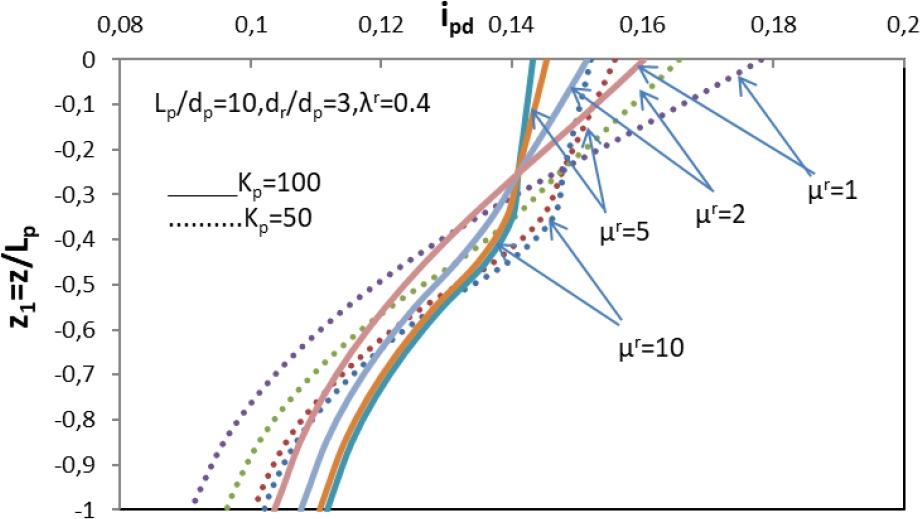Variation of SIF for any depth, ipd, with normalized depth, z1=z/Lp–effect of stiffness factor, μr, on homogeneous GP–raft and partially strengthened GP–raft (Lp/dp=10, dr/dp=3, λr=0.4).

The variation of SIF, ipd, of a GP with normalized depth, z1 = z/Lp, is shown in Fig. 17 with the effect of comparative length λr of strengthening from top of GP for Lp/dp=10, Kp=50, dr/dp=3, and μr= 4. For comparative length of strengthening from top of GP, λr = 0.1, 0.2, 0.3, and 0.4, the values of SIF, ipd, for top are, respectively, 0.172, 0.167, 0.161, and 0.157 for percentage decreases of 2.9, 6.3, and 8.7. SIF, ipd, decreases rapidly with depth in the lower region, i.e., in unstrengthened portion of GP. For higher value of λ, the value of ipd is maximum due to stresses transferred to the base with the length of strengthening.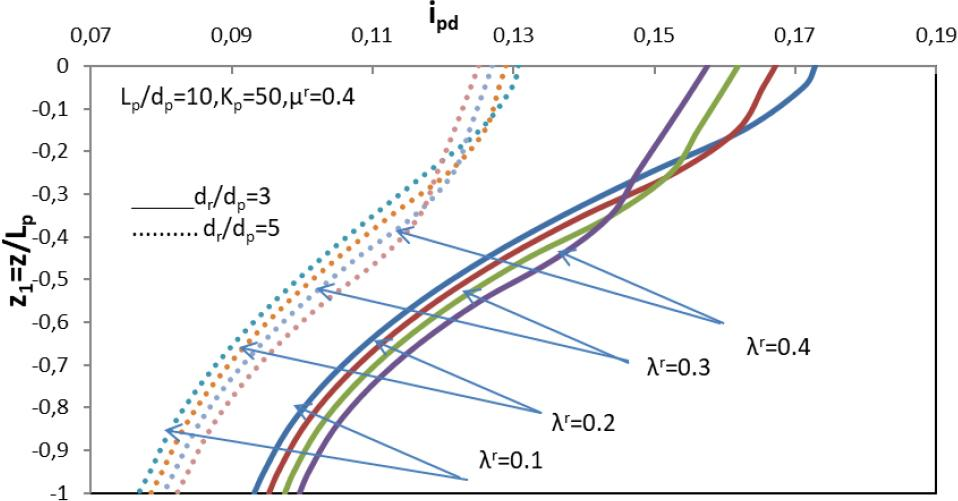Variation of SIF for any depth, ipd, with normalized depth, z1=z/Lp – effect of comparative length λr of strengthening from top of GP on partially strengthened GP–raft foundation (Lp/dp=10, Kp=50, μr=0.4).

Variations of contact pressures, pr* = (Fr/q), with normalized distance from the center of raft R*=r/dp, with stiffness factor, μr. Effects of vertical and radial compatibility of displacement on a strengthened GP raft and raft alone are shown in Fig. 18. The contact pressure distribution pattern for partially strengthened piled raft is very similar to that of the raft alone with the normal stress beneath a rigid raft increasing with distance from the center and tending to very high values at the edge of the raft. The percentage reductions in the contact pressure for Lp/dp=10, dr/dp=3, Kp= 50, and μr = 10 for vertical and considering radial with vertical compatibility in comparison to the value for a solid raft are, respectively, 23.28% and 14.28% near the edge of raft.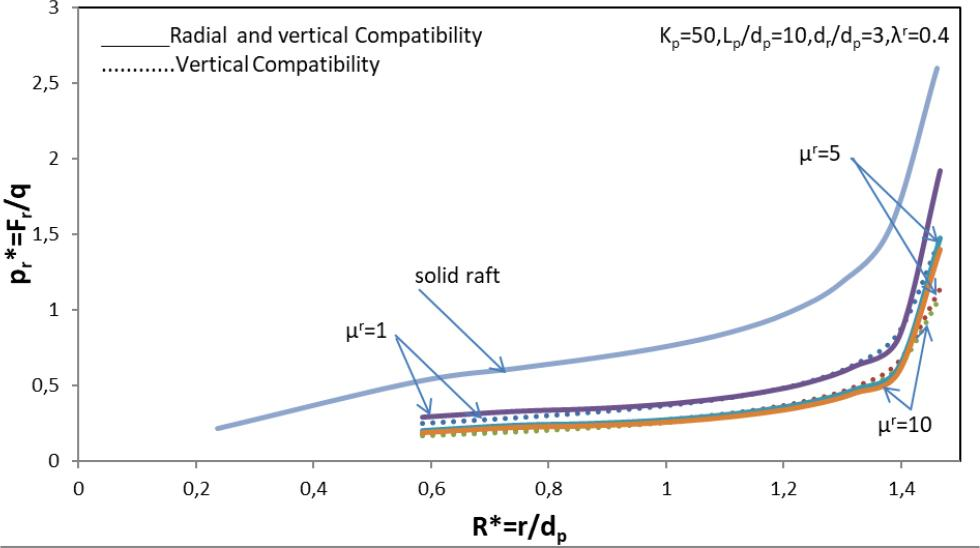Variation of contact pressure (pr* =Fr/q) with normalized distance from the center of raft R*=r/dp–effect of strengthening factor, μr, and vertical and radial compatibility of displacement on a partially strengthened GP–raft and solid raft (Kp =50, Lp/dp=10, dr/dp=3, λr=0.4).

The contact pressure distribution at the raft–soil interface, pr*=(Fr/q), with normalized distance from the center of raft, R* = r/dp, can be seen in Fig. 19 for Lp/dp=10, μr=4, λr=0.4, and dr/dp = 3 and 5. The percentage reduction in contact pressure for Kp = 50 and 100 for dr/dp=3 and 5 in comparison to the values for Kp =30 are 8.6% and 21.7% near the pile and 5% and 15% at the edge of pile, respectively. For smaller size (dr/dp =3) of raft, the stresses are about 35.12% lower than those for the larger size (dr/dp =5) at the edge of raft for Kp= 100. The magnitude of normal stresses on raft decreases with increase in the strengthening of GP.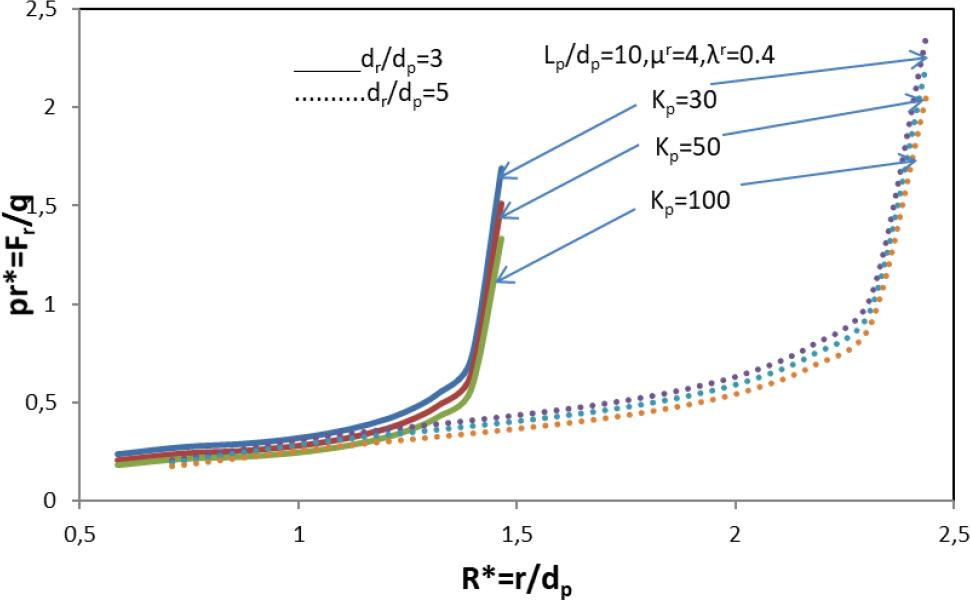Variation of contact pressure (pr* =Fr/q) with normalized distance from the center of raft R*=r/dp–effect of comparative strengthening, Kp, of GP and comparative size of raft, dr/dp, on partially strengthened GP–raft foundation (Lp/dp=10, μr=4, λr=0.4).

Fig. 20 shows the deviation of percentage pile load, (Fp/F) ×100, and raft load, (Fp/F) ×100, with comparative strengthening, Kp, of GP with the influence of strengthening factor, μr, on a partially strengthen granular piled raft for Lp/dp=10, dr/dp=3, λr=0.4. As can be expected, the percentage load of GP rises with increases of comparative strengthening factor, μr, and vice versa raft load decreases. With the increase in strengthening factor μr=1–5, the percentage load sharing by pile is increased from 20 to 41.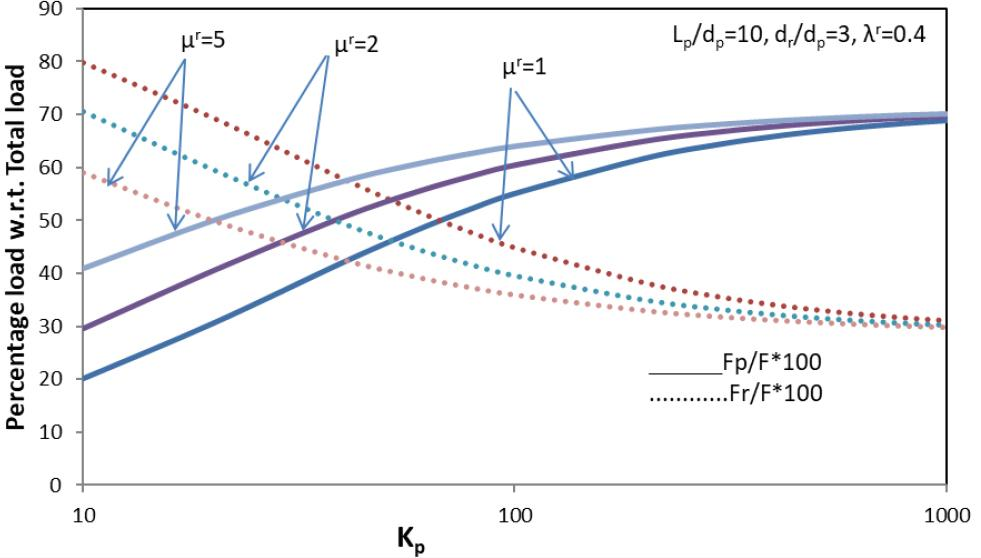Variation of percentage load w. r. t. total load with the comparative strengthening of GP, Kp – effect of comparative length of strengthening of GP, μr, on a partially strengthen GP–raft foundation (Lp/dp=10, dr/dp=3,λr=0.4).

Variation of percentage pile load, (Fp/F) ×100, and raft load, (Fr/F) × 100, with comparative stiffness, Kp, with the influence of comparative size of raft, dr/dp, on a GP raft for Lp/dp=10, μr=4, λr=0.4 is shown in Fig. 21. The percentage load of GP decreases with the increase of the comparative size of raft, dr/dp, and vice versa raft load increases. As the size of raft increases, the load on raft increases.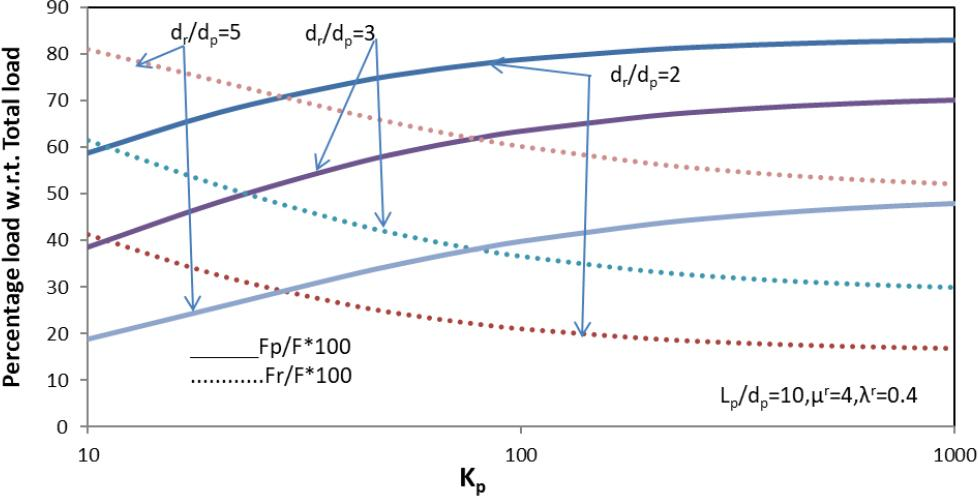Variation of percentage load w. r. t. total load with the comparative strengthening of GP, Kp– effect of comparative length of GP, dr/dp, on a partially strengthen GP–raft foundation (Lp/dp=10,μr=4,λr=0.4).

The variation of percentage raft load, (Fr/F) × 100, with comparative strengthening of GP, Kp, is shown in Fig. 22 with the effect of the comparative length of strengthening, λr, on a partially strengthened granular piled raft. The percentage raft load decreases with the rise in comparative length of strengthening, λr. For Lp/dp=10, dr/dp=3, μr=4, and λr=0.2, 0.3, and 0.4, the values of percentage raft load decrease to 66, 63, and 61 correspondingly. The percentage raft load decreases from 66 to 61 for an increase in comparative length of strengthening, λr, from 0.2 to 0.4.

Conclusions

Based on the finite difference technique and elastic continuum approach, new pile displacement matrix is formulated in current study. The following conclusions are drawn from this study:

– The differences in the values of SIF, ip, obtained from the two analyses with and without radial compatibility of displacement are in the range of 1–1.5%. Thus, the consideration of radial displacement compatibility of GP in the analysis does not influence the SIF significantly.

– The decrease in the SIF with the increase of strengthening factor, μr, and comparative length of strengthening from top of GP, λr. Decrease in the SIF from μ= 1 to 5 is about 13.83% and from μ= 5 to 10 is only 4% for L/d=10, Kp = 10, D/d = 3, and λ = 0.4 which implies that the effect is more pronounced in the range of stiffness factor μ =1–5. Further strengthening of GP in the top portion does not make a significant change in the settlement reduction.

– The radial displacement impact factor, ir, reduces with increasing strengthening parameters, μr and λr, thus producing the beneficial effect of strengthening, which means reducing the bulging in terms of radial displacement impact factor, ir, in top portion of GP. Maximum radial displacement is observed in (0–3) Lp length of GP.

– The shear stresses along GP–soil interface are negative in its top region due to influence of normal stresses transferred by the raft, i.e., an effect similar to down-drag for partially strengthened granular piled raft improved along the GP. Due to strengthening in the upper portion of GP, shear stresses increase along the pile length.

– Normalized radial stresses tendto increase with the strengthening of GP. Radial stresses tend to decrease in upper portion with the size of raft in upper portion and increase in lower part of GP because of higher distance of raft edge stresses.

– The percentage reduction in the contact pressure at the raft–soil interface in comparison to the value of a solid raft is about 14% near the edge of raft for Lp/dp = 10, dr/dp = 3, μr = 10, and Kp = 50.

– The percentage load transferred to the pile increases with the increase in strengthening parameters and comparative strengthening of GP, Kp, and vice versa percentage raft load decreases as it reflects true behavior of piled raft foundation system.

Burland, J. B. (1977). Behaviour of foundations and structures on soft ground. Proc. 9th ICSMFE, 1977, 2, 495–546. BurlandJ. B. 1977 Behaviour of foundations and structures on soft ground Proc. 9th ICSMFE, 1977 2 495 546 Search in Google Scholar

Poulos, H. G. (1968). Analysis of the settlement of pile groups. Geotechnique, 18(4), 449–471. PoulosH. G. 1968 Analysis of the settlement of pile groups Geotechnique 18 4 449 471 10.1680/geot.1968.18.4.449 Search in Google Scholar

Randolph, M. F. (1983). Design of Piled Raft Foundations. Cambridge University. Eng. Depart. Research Report, Soils TR143, Cambridge. RandolphM. F. 1983 Design of Piled Raft Foundations. Cambridge University Eng. Depart. Research Report, Soils TR143, Cambridge Search in Google Scholar

Huang, M., Liang, F., & Li, Z. (2007). Recent advances in the analysis of pile foundation in China. In Advances in deep foundations (pp. 127–136). CRC Press. HuangM. LiangF. LiZ. 2007 Recent advances in the analysis of pile foundation in China In Advances in deep foundations 127 136 CRC Press Search in Google Scholar

Poulos, H.G. (2001). Piled raft foundations: design and applications. Geotechnique, 51(2), 95–113. PoulosH.G. 2001 Piled raft foundations: design and applications Geotechnique 51 2 95 113 10.1680/geot.2001.51.2.95 Search in Google Scholar

Liang, F. Y., Chen, L. Z., & Shi, X. G. (2003). Numerical analysis of composite piled raft with cushion subjected to vertical load. Computers and Geotechnics, 30(6), 443–453. LiangF. Y. ChenL. Z. ShiX. G. 2003 Numerical analysis of composite piled raft with cushion subjected to vertical load Computers and Geotechnics 30 6 443 453 10.1016/S0266-352X(03)00057-0 Search in Google Scholar

Liang, F., Li, J., & Chen, L. (2006). Optimization of composite piled raft foundation with varied rigidity of cushion. In Foundation Analysis and Design: Innovative Methods (pp. 29–34). LiangF. LiJ. ChenL. 2006 Optimization of composite piled raft foundation with varied rigidity of cushion In Foundation Analysis and Design: Innovative Methods 29 34 10.1061/40865(197)3 Search in Google Scholar

Eslami, A., & Malekshah, S. S. (2011). Analysis of non-connected piled raft foundations (NCPRF) with cushion by finite element method. Computational Methods in Civil Engineering, 2(2). EslamiA. MalekshahS. S. 2011 Analysis of non-connected piled raft foundations (NCPRF) with cushion by finite element method Computational Methods in Civil Engineering 2 2 Search in Google Scholar

Tradigo, F., Pisanò, F., Di Prisco, C., & Mussi, A. (2015). Non-linear soil–structure interaction in disconnected piled raft foundations. Computers and Geotechnics, 63, 121–134. TradigoF. PisanòF. Di PriscoC. MussiA. 2015 Non-linear soil–structure interaction in disconnected piled raft foundations Computers and Geotechnics 63 121 134 10.1016/j.compgeo.2014.08.014 Search in Google Scholar

Tradigo, F., Pisanò, F., & di Prisco, C. (2016). On the use of embedded pile elements for the numerical analysis of disconnected piled rafts. Computers and Geotechnics, 72, 89–99. TradigoF. PisanòF. di PriscoC. 2016 On the use of embedded pile elements for the numerical analysis of disconnected piled rafts Computers and Geotechnics 72 89 99 10.1016/j.compgeo.2015.11.005 Search in Google Scholar

Sharma, V. J., Vasanvala, S. A., & Solanki, C. H. (2014). Behaviour of load-bearing components of a cushioned composite piled raft foundation under axial loading. Slovak Journal of Civil Engineering, 22(4), 25–34. SharmaV. J. VasanvalaS. A. SolankiC. H. 2014 Behaviour of load-bearing components of a cushioned composite piled raft foundation under axial loading Slovak Journal of Civil Engineering 22 4 25 34 10.2478/sjce-2014-0020 Search in Google Scholar

Eid, H. T., & Shehada, A. A. (2015). Estimating the elastic settlement of piled foundations on rock. International Journal of Geomechanics, 15(3), 04014059. EidH. T. ShehadaA. A. 2015 Estimating the elastic settlement of piled foundations on rock International Journal of Geomechanics 15 3 04014059 10.1061/(ASCE)GM.1943-5622.0000376 Search in Google Scholar

Huang, M., Liang, F., & Jiang, J. (2011). A simplified nonlinear analysis method for piled raft foundation in layered soils under vertical loading. Computers and Geotechnics, 38(7), 875–882. HuangM. LiangF. JiangJ. 2011 A simplified nonlinear analysis method for piled raft foundation in layered soils under vertical loading Computers and Geotechnics 38 7 875 882 10.1016/j.compgeo.2011.06.002 Search in Google Scholar

Kuwabara, F. (1989). An elastic analysis for piled raft foundations in a homogeneous soil. Soils and foundations, 29(1), 82–92. KuwabaraF. 1989 An elastic analysis for piled raft foundations in a homogeneous soil Soils and foundations 29 1 82 92 10.3208/sandf1972.29.82 Search in Google Scholar

Lee, S., & Moon, J. S. (2017). Effect of interactions between piled raft components and soil on behavior of piled raft foundation. KSCE Journal of Civil Engineering, 21(1), 243–252. LeeS. MoonJ. S. 2017 Effect of interactions between piled raft components and soil on behavior of piled raft foundation KSCE Journal of Civil Engineering 21 1 243 252 10.1007/s12205-016-0880-z Search in Google Scholar

Luo, R., Yang, M., & Li, W. (2018). Normalized settlement of piled raft in homogeneous clay. Computers and Geotechnics, 103, 165–178. LuoR. YangM. LiW. 2018 Normalized settlement of piled raft in homogeneous clay Computers and Geotechnics 103 165 178 10.1016/j.compgeo.2018.07.023 Search in Google Scholar

Maharaj, D. K., & Gandhi, S. R. (2004). Non-linear finite element analysis of piled-raft foundations. Proceedings of the Institution of Civil Engineers-Geotechnical Engineering, 157(3), 107–113. MaharajD. K. GandhiS. R. 2004 Non-linear finite element analysis of piled-raft foundations Proceedings of the Institution of Civil Engineers-Geotechnical Engineering 157 3 107 113 10.1680/geng.2004.157.3.107 Search in Google Scholar

Shen, W. Y., Chow, Y. K., & Yong, K. Y. (2000). A variational approach for the analysis of pile group–pile cap interaction. Geotechnique, 50(4), 349–357. ShenW. Y. ChowY. K. YongK. Y. 2000 A variational approach for the analysis of pile group–pile cap interaction Geotechnique 50 4 349 357 10.1680/geot.2000.50.4.349 Search in Google Scholar

Mindlin, R. D. (1936). Force at a point in the interior of a semi-infinite solid. physics, 7(5), 195–202. MindlinR. D. 1936 Force at a point in the interior of a semi-infinite solid physics 7 5 195 202 10.21236/AD0012375 Search in Google Scholar

Sharma, J. K. (1999). Analysis and settlement of granular pile (s)-single, in group and with raft (Doctoral dissertation, Ph. D. Thesis, IIT, Kanpur, 408). SharmaJ. K. 1999 Analysis and settlement of granular pile (s)-single, in group and with raft (Doctoral dissertation, Ph. D. Thesis, IIT, Kanpur, 408). Search in Google Scholar

Madhav, M. R., Sharma, J. K., & Sivakumar, V. (2009). Settlement of and load distribution in a granular piled raft. Geomechanics and Engineering, 1(1), 97–112. MadhavM. R. SharmaJ. K. SivakumarV. 2009 Settlement of and load distribution in a granular piled raft Geomechanics and Engineering 1 1 97 112 10.12989/gae.2009.1.1.097 Search in Google Scholar

Empfohlene Artikel von Trend MD